# FOUNDATION Physics Hydrostatistics

## Hydrostatistics Synopsis

Synopsis

Pascal’s Law

• Pascal’s law explains how pressure is transmitted in liquids.
• It states that, “The pressure exerted anywhere in a confined liquid is transmitted equally and undiminished in all the directions throughout the liquid”.
• Pascal’s law can be demonstrated with the help of the following experiment:
o Take a glass flask with narrow tubes coming out from its sides and bottom. The flask is provided with an air-tight piston at its mouth.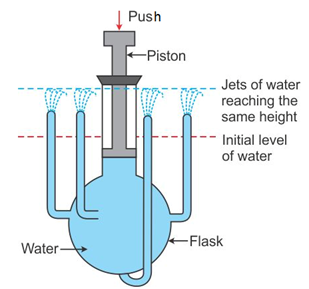o Fill the flask with water. The water in each tube will be at the same level.
o The initial level of water in each tube is shown by the dotted red line.
o Now, push the piston down into the flask gently. It is observed that jets of water rise out from each tube, reaching the same height which is shown by the dotted blue line.

Application of Pascal’s Law

• Hydraulic press, hydraulic jack and hydraulic brakes work on Pascal’s law of transmission of pressure.
• The principle of the working of these hydraulic machines is, “A small force applied on a smaller piston is transmitted to produce a large force on the bigger piston”.
• Consider two cylindrical vessels P and Q connected by a horizontal tube R. The vessels contain a liquid, and they are provided with water-tight pistons A and B.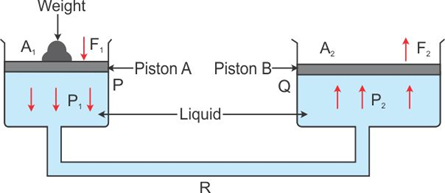• The vessel P is of smaller diameter than the vessel Q. Let the area of cross section of the vessel P be A1 and that of the vessel Q be A2. A weight is placed on the piston A. It exerts a force F1 on the piston A. Therefore, the pressure applied on the piston A is• According to Pascal’s law, the pressure exerted on piston A is transmitted through the liquid to piston B. Therefore, the upward pressure on B is• Hence, the force on piston B is
F2 = Pressure on piston B isTherefore, we have• Since, A2 > A1, we get F2 > F1.
Buoyancy
• Whenever an object is immersed in water or any other liquid, it appears to lose some weight and feels lighter.
• The weight of the object exerts a force on the liquid. The liquid also exerts a certain amount of force on the object in the upwards direction. This force is called buoyant force.
• The tendency of a liquid to exert an upward force on any object immersed in it is called buoyancy.
• The upward force exerted by a liquid is also called upthrust.

Why Objects Float or Sink when Placed on a Surface of Water
• If the downward gravitational force of an object is greater than the upward buoyant force of water, then the object sinks. However, if the gravitational force is less, then the object floats.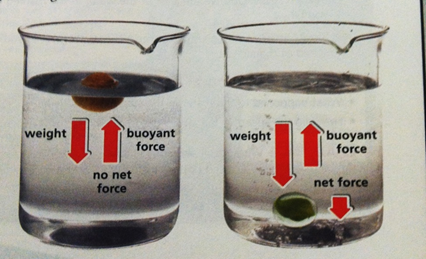The light plastic ball floats, while the heavy marble sinks.

• Objects with a density lesser than water float on water, and objects with a density greater than water sink in it.
• The magnitude of buoyant force acting on an object immersed in a liquid depends on:
o Volume of object immersed in the liquid. This is because the density changes with volume.
o Density of the liquid.

Archimede’s Principle

• When an object is immersed wholly or partially in a fluid, it experiences an upward force that is equal to the weight of the fluid displaced by it.
Buoyant force acting on an object = weitght of fluid displaced by that object
• Gases however exert a very negligible buoyant force on the objects placed in them.• A boat floats on water due to the Archimedes’ principle. The boat displaces water equal to its own weight. This displaced water exerts buoyant force to balance the weight of the boat and keep it floating on water.

Applications of the Archimedes’ Principle

• The Archimedes’ principle is used in determining the relative density of a substance.
• Hydrometers used to determine the density of liquids are based on the Archimedes’ principle.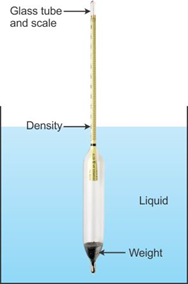• Lactometers used to determine the purity of milk are based on the Archimedes’ principle.
• The Archimedes’ principle is also used in designing ships and submarines.

Density

• The density of a substance is defined as mass per unit volume.
• Different substances have different densities.
• It is a scalar quantity and is represented by the Greek letter ρ.•  Its SI unit is kg m−3 and its CGS unit is g cm−3.Most substances expand on heating and contract on cooling.
• Thus, the density of a substance increases with decrease in temperature and decreases with increase in temperature.

Relative Density (RD)

• It is convenient to express the density of a substance in comparison to the density of water.
• The relative density of a substance is the ratio of its density to that of water at 4°C.• It is also given as the ratio of the mass of the substance to the mass of equal volume of water at 4°C.• Because it is a ratio of similar quantities, it has no units.
• In the SI system, the density of water is 1000 kg m−3. Thus, we have∴ Density in kg m-3 = 1000 R.D
• Now, the density of water in the CGS system is 1 g cm−3. Thus, we have∴ Density in g cm-3 = R.D

Distinction between Density and Relative Density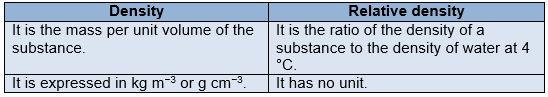Relative Density of a Solid by Archimedes’ Principle

• From the definition of relative density, we have